# 6 estimation hypothesis testing t test

31. Oct 2018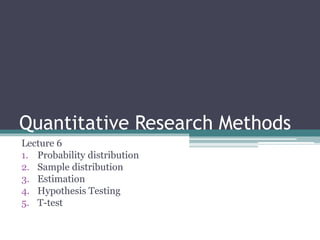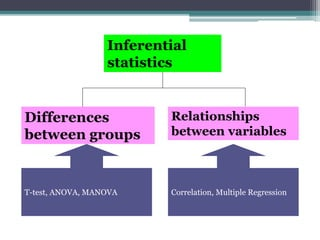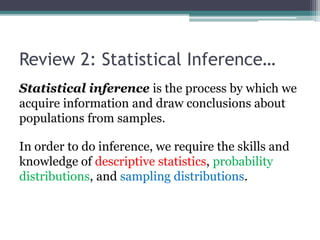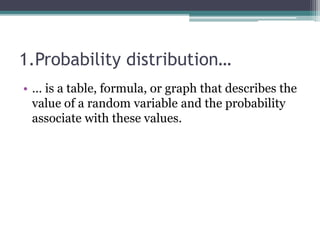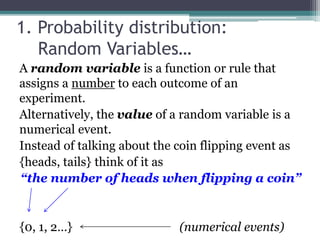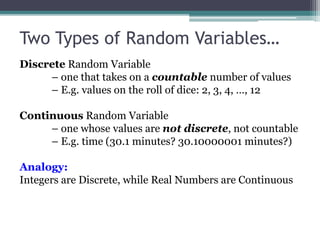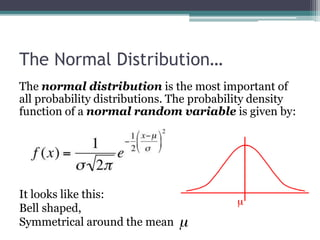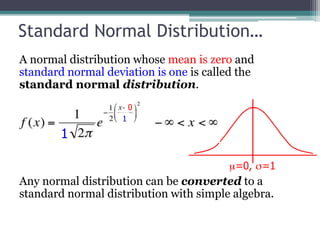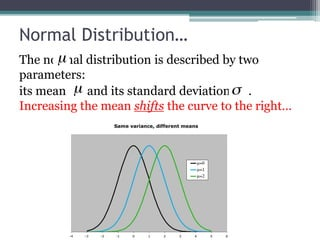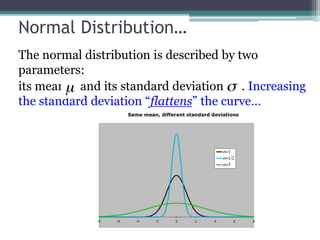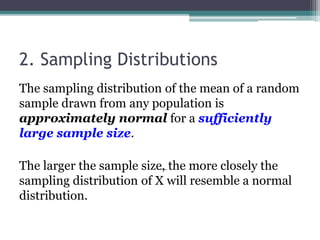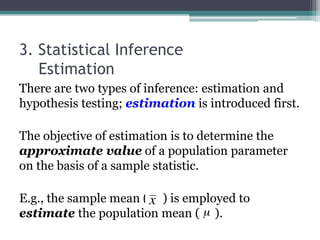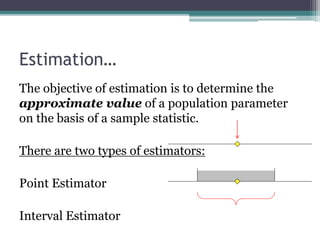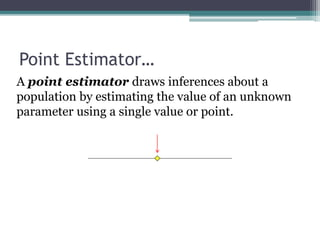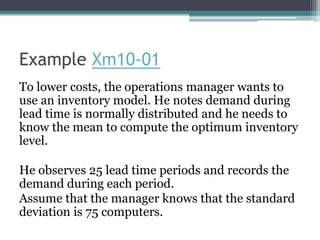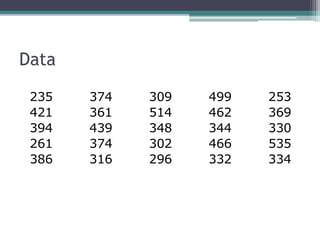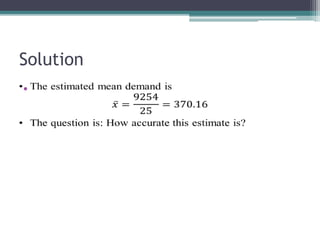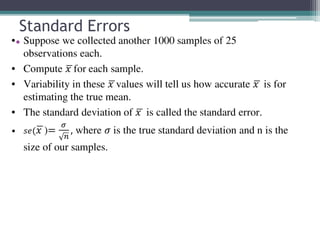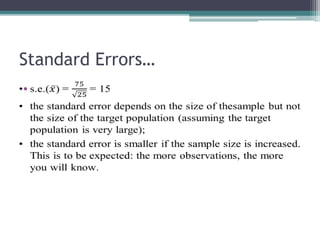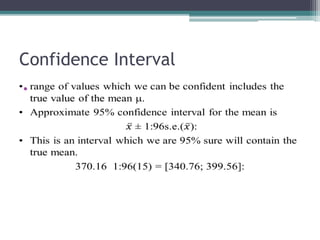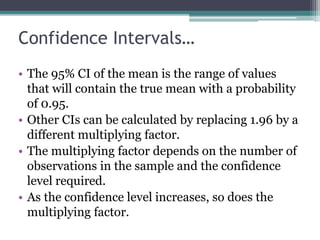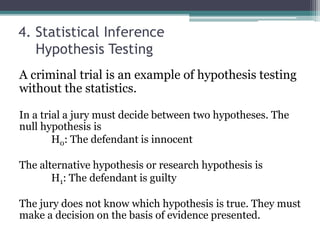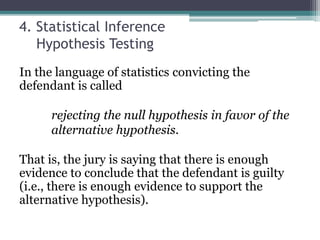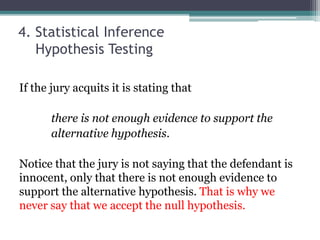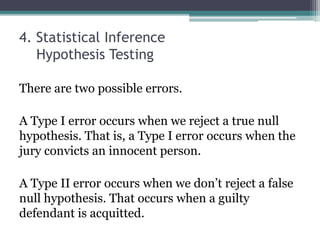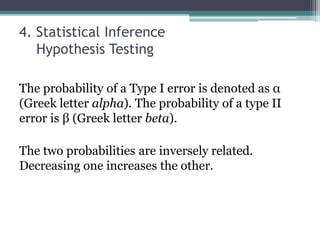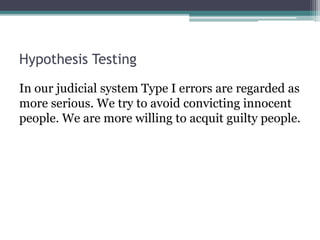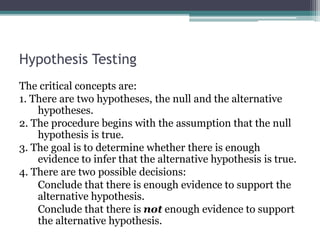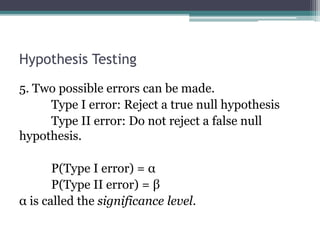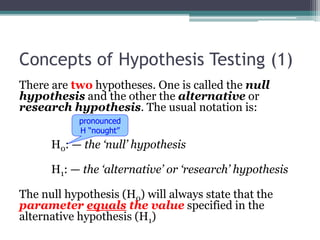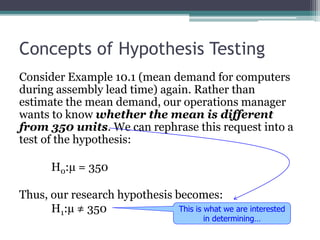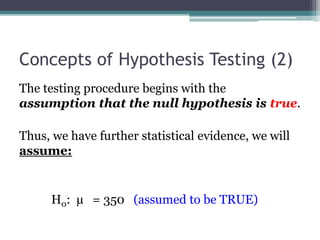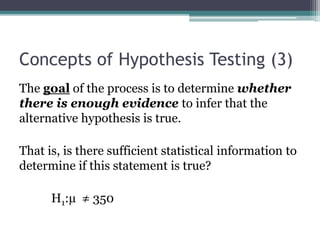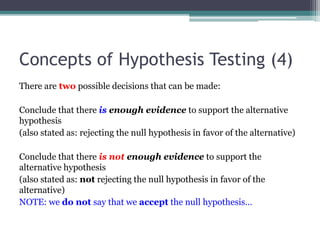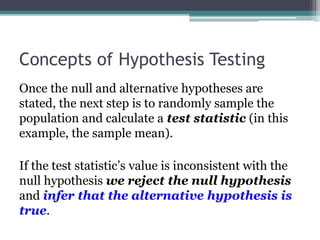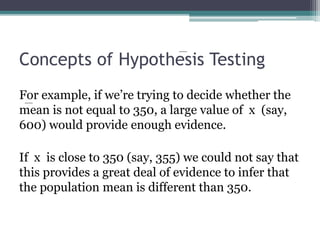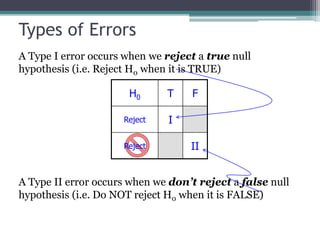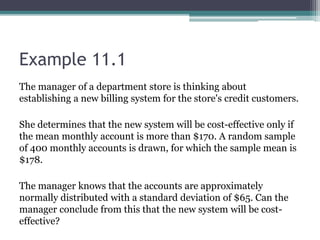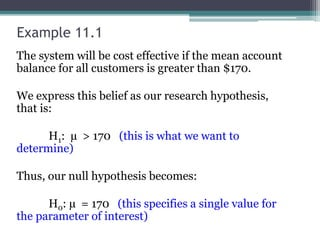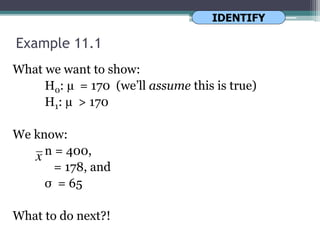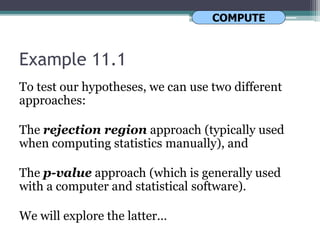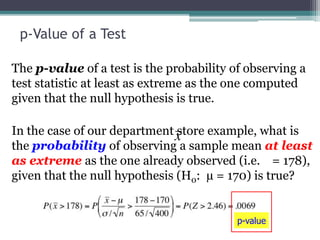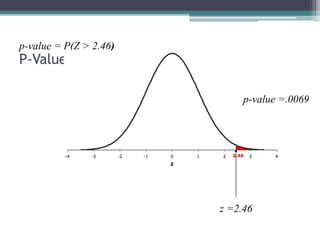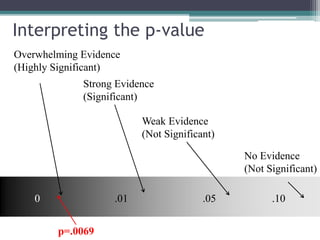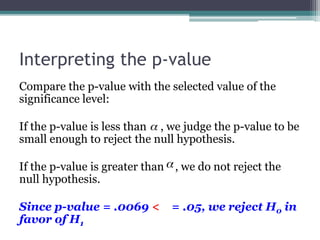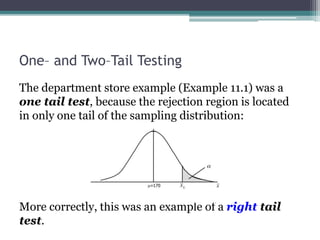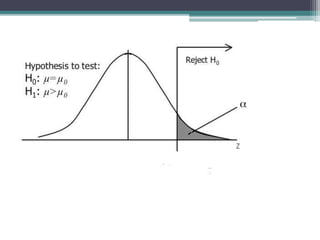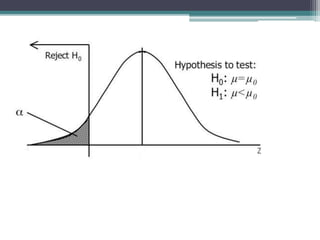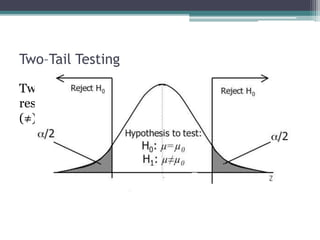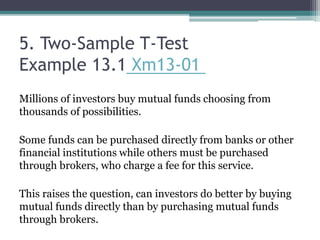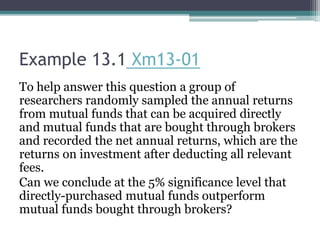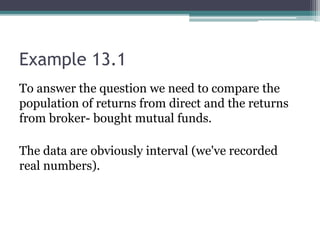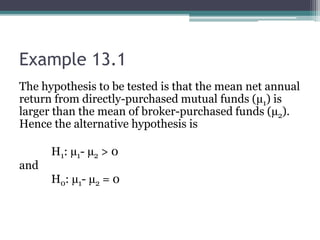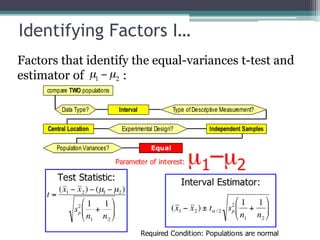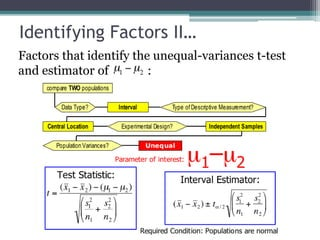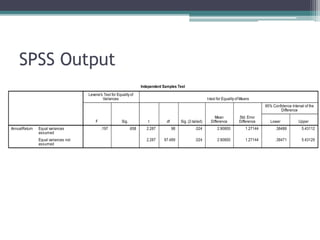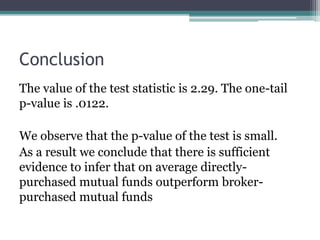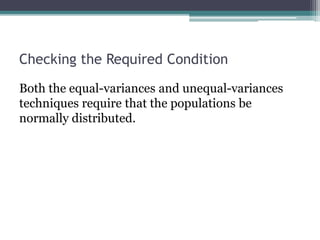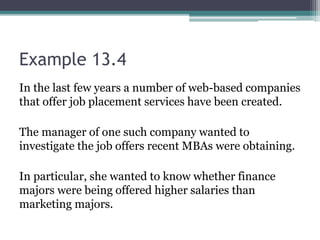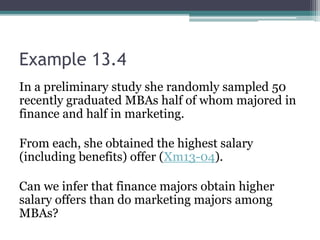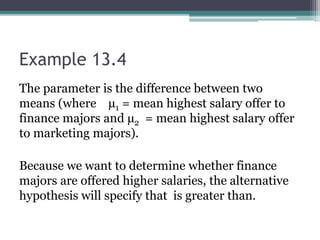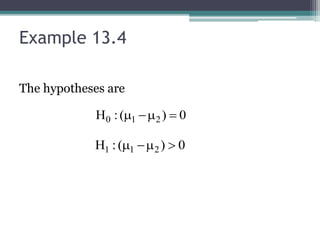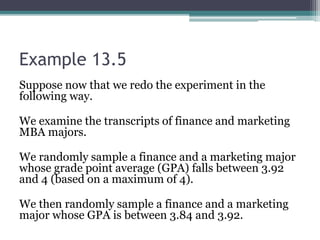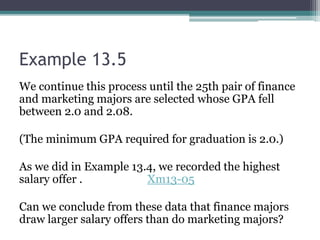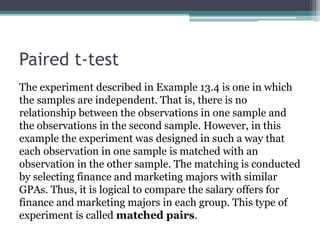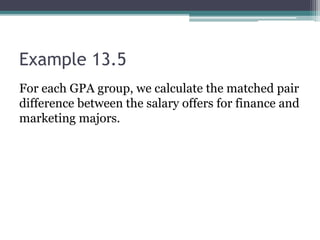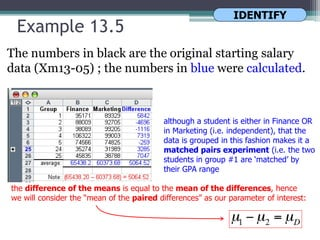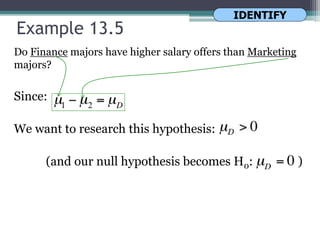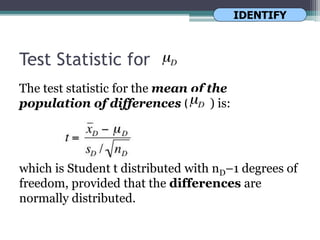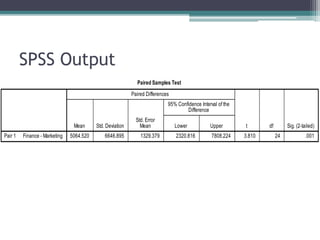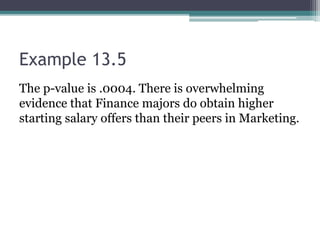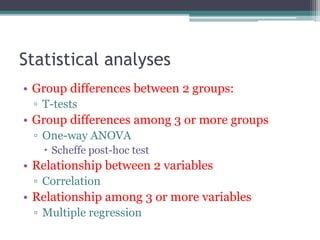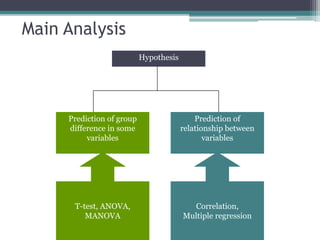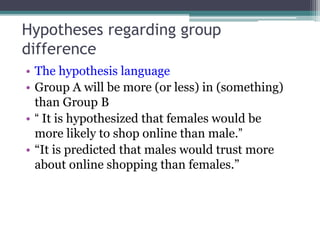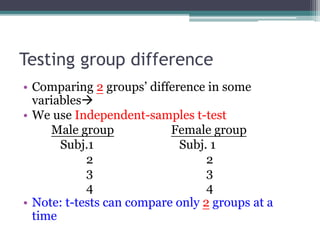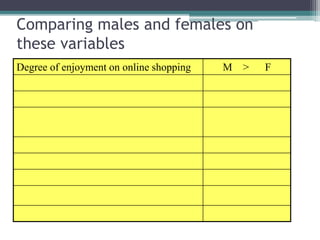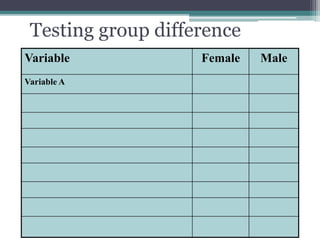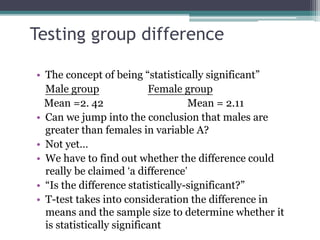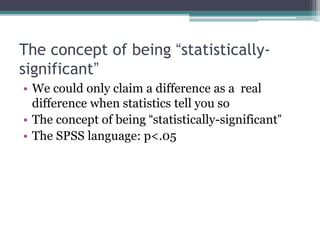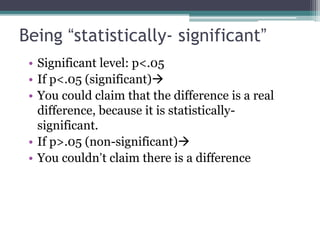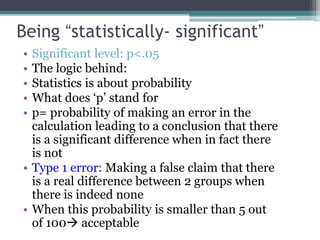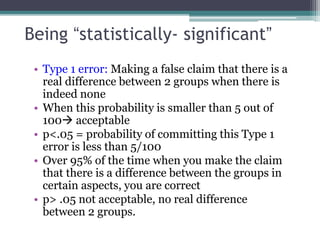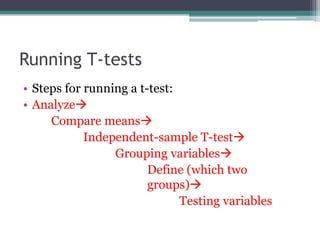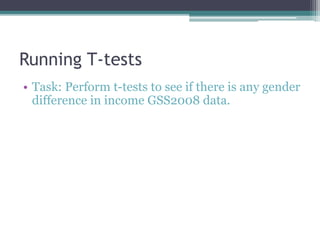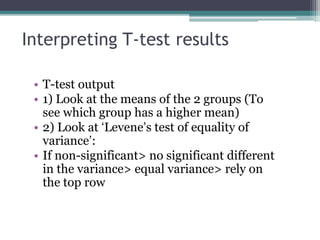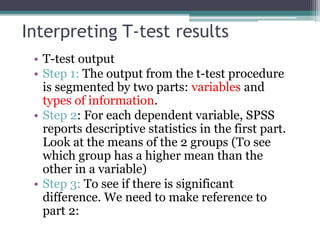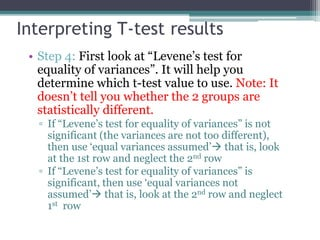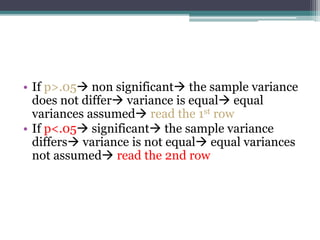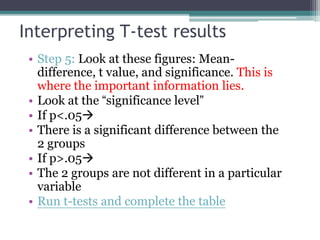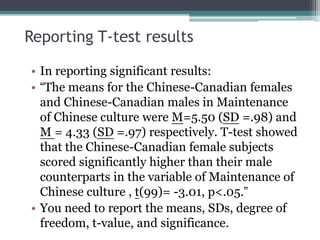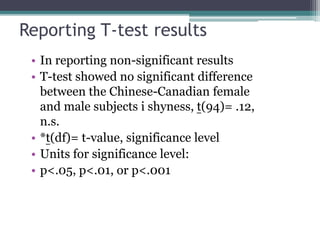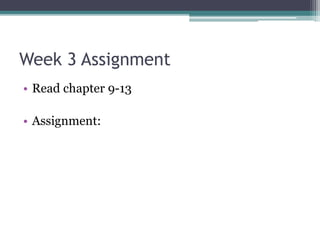1 von 96

### 6 estimation hypothesis testing t test

• 1. Quantitative Research Methods Lecture 6 1. Probability distribution 2. Sample distribution 3. Estimation 4. Hypothesis Testing 5. T-test
• 2. Inferential statistics Differences between groups Relationships between variables T-test, ANOVA, MANOVA Correlation, Multiple Regression
• 3. Review 2: Statistical Inference… Statistical inference is the process by which we acquire information and draw conclusions about populations from samples. In order to do inference, we require the skills and knowledge of descriptive statistics, probability distributions, and sampling distributions.
• 4. 1.Probability distribution… • … is a table, formula, or graph that describes the value of a random variable and the probability associate with these values.
• 5. 1. Probability distribution: Random Variables… A random variable is a function or rule that assigns a number to each outcome of an experiment. Alternatively, the value of a random variable is a numerical event. Instead of talking about the coin flipping event as {heads, tails} think of it as “the number of heads when flipping a coin” {0, 1, 2…} (numerical events)
• 6. Two Types of Random Variables… Discrete Random Variable – one that takes on a countable number of values – E.g. values on the roll of dice: 2, 3, 4, …, 12 Continuous Random Variable – one whose values are not discrete, not countable – E.g. time (30.1 minutes? 30.10000001 minutes?) Analogy: Integers are Discrete, while Real Numbers are Continuous
• 7. The Normal Distribution… The normal distribution is the most important of all probability distributions. The probability density function of a normal random variable is given by: It looks like this: Bell shaped, Symmetrical around the mean
• 8. Standard Normal Distribution… A normal distribution whose mean is zero and standard normal deviation is one is called the standard normal distribution. Any normal distribution can be converted to a standard normal distribution with simple algebra. 0 1 1
• 9. Normal Distribution… The normal distribution is described by two parameters: its mean and its standard deviation . Increasing the mean shifts the curve to the right…
• 10. Normal Distribution… The normal distribution is described by two parameters: its mean and its standard deviation . Increasing the standard deviation “flattens” the curve…
• 11. 2. Sampling Distributions The sampling distribution of the mean of a random sample drawn from any population is approximately normal for a sufficiently large sample size. The larger the sample size, the more closely the sampling distribution of X will resemble a normal distribution.
• 12. 3. Statistical Inference Estimation There are two types of inference: estimation and hypothesis testing; estimation is introduced first. The objective of estimation is to determine the approximate value of a population parameter on the basis of a sample statistic. E.g., the sample mean ( ) is employed to estimate the population mean ( ).
• 13. Estimation… The objective of estimation is to determine the approximate value of a population parameter on the basis of a sample statistic. There are two types of estimators: Point Estimator Interval Estimator
• 14. Point Estimator… A point estimator draws inferences about a population by estimating the value of an unknown parameter using a single value or point.
• 15. Example Xm10-01 To lower costs, the operations manager wants to use an inventory model. He notes demand during lead time is normally distributed and he needs to know the mean to compute the optimum inventory level. He observes 25 lead time periods and records the demand during each period. Assume that the manager knows that the standard deviation is 75 computers.
• 16. Data 235 374 309 499 253 421 361 514 462 369 394 439 348 344 330 261 374 302 466 535 386 316 296 332 334
• 17. Solution •
• 18. Standard Errors •
• 19. Standard Errors… •
• 20. Confidence Interval •
• 21. Confidence Intervals… • The 95% CI of the mean is the range of values that will contain the true mean with a probability of 0.95. • Other CIs can be calculated by replacing 1.96 by a different multiplying factor. • The multiplying factor depends on the number of observations in the sample and the confidence level required. • As the confidence level increases, so does the multiplying factor.
• 22. 4. Statistical Inference Hypothesis Testing A criminal trial is an example of hypothesis testing without the statistics. In a trial a jury must decide between two hypotheses. The null hypothesis is H0: The defendant is innocent The alternative hypothesis or research hypothesis is H1: The defendant is guilty The jury does not know which hypothesis is true. They must make a decision on the basis of evidence presented.
• 23. 4. Statistical Inference Hypothesis Testing In the language of statistics convicting the defendant is called rejecting the null hypothesis in favor of the alternative hypothesis. That is, the jury is saying that there is enough evidence to conclude that the defendant is guilty (i.e., there is enough evidence to support the alternative hypothesis).
• 24. 4. Statistical Inference Hypothesis Testing If the jury acquits it is stating that there is not enough evidence to support the alternative hypothesis. Notice that the jury is not saying that the defendant is innocent, only that there is not enough evidence to support the alternative hypothesis. That is why we never say that we accept the null hypothesis.
• 25. 4. Statistical Inference Hypothesis Testing There are two possible errors. A Type I error occurs when we reject a true null hypothesis. That is, a Type I error occurs when the jury convicts an innocent person. A Type II error occurs when we don’t reject a false null hypothesis. That occurs when a guilty defendant is acquitted.
• 26. 4. Statistical Inference Hypothesis Testing The probability of a Type I error is denoted as α (Greek letter alpha). The probability of a type II error is β (Greek letter beta). The two probabilities are inversely related. Decreasing one increases the other.
• 27. Hypothesis Testing In our judicial system Type I errors are regarded as more serious. We try to avoid convicting innocent people. We are more willing to acquit guilty people.
• 28. Hypothesis Testing The critical concepts are: 1. There are two hypotheses, the null and the alternative hypotheses. 2. The procedure begins with the assumption that the null hypothesis is true. 3. The goal is to determine whether there is enough evidence to infer that the alternative hypothesis is true. 4. There are two possible decisions: Conclude that there is enough evidence to support the alternative hypothesis. Conclude that there is not enough evidence to support the alternative hypothesis.
• 29. Hypothesis Testing 5. Two possible errors can be made. Type I error: Reject a true null hypothesis Type II error: Do not reject a false null hypothesis. P(Type I error) = α P(Type II error) = β α is called the significance level.
• 30. Concepts of Hypothesis Testing (1) There are two hypotheses. One is called the null hypothesis and the other the alternative or research hypothesis. The usual notation is: H0: — the ‘null’ hypothesis H1: — the ‘alternative’ or ‘research’ hypothesis The null hypothesis (H0) will always state that the parameter equals the value specified in the alternative hypothesis (H1) pronounced H “nought”
• 31. Concepts of Hypothesis Testing Consider Example 10.1 (mean demand for computers during assembly lead time) again. Rather than estimate the mean demand, our operations manager wants to know whether the mean is different from 350 units. We can rephrase this request into a test of the hypothesis: H0:µ = 350 Thus, our research hypothesis becomes: H1:µ ≠ 350 This is what we are interested in determining…
• 32. Concepts of Hypothesis Testing (2) The testing procedure begins with the assumption that the null hypothesis is true. Thus, we have further statistical evidence, we will assume: H0: µ = 350 (assumed to be TRUE)
• 33. Concepts of Hypothesis Testing (3) The goal of the process is to determine whether there is enough evidence to infer that the alternative hypothesis is true. That is, is there sufficient statistical information to determine if this statement is true? H1:µ ≠ 350
• 34. Concepts of Hypothesis Testing (4) There are two possible decisions that can be made: Conclude that there is enough evidence to support the alternative hypothesis (also stated as: rejecting the null hypothesis in favor of the alternative) Conclude that there is not enough evidence to support the alternative hypothesis (also stated as: not rejecting the null hypothesis in favor of the alternative) NOTE: we do not say that we accept the null hypothesis…
• 35. Concepts of Hypothesis Testing Once the null and alternative hypotheses are stated, the next step is to randomly sample the population and calculate a test statistic (in this example, the sample mean). If the test statistic’s value is inconsistent with the null hypothesis we reject the null hypothesis and infer that the alternative hypothesis is true.
• 36. Concepts of Hypothesis Testing For example, if we’re trying to decide whether the mean is not equal to 350, a large value of x (say, 600) would provide enough evidence. If x is close to 350 (say, 355) we could not say that this provides a great deal of evidence to infer that the population mean is different than 350.
• 37. Types of Errors A Type I error occurs when we reject a true null hypothesis (i.e. Reject H0 when it is TRUE) A Type II error occurs when we don’t reject a false null hypothesis (i.e. Do NOT reject H0 when it is FALSE) H0 T F Reject I Reject II
• 38. Example 11.1 The manager of a department store is thinking about establishing a new billing system for the store's credit customers. She determines that the new system will be cost-effective only if the mean monthly account is more than \$170. A random sample of 400 monthly accounts is drawn, for which the sample mean is \$178. The manager knows that the accounts are approximately normally distributed with a standard deviation of \$65. Can the manager conclude from this that the new system will be cost- effective?
• 39. Example 11.1 The system will be cost effective if the mean account balance for all customers is greater than \$170. We express this belief as our research hypothesis, that is: H1: µ > 170 (this is what we want to determine) Thus, our null hypothesis becomes: H0: µ = 170 (this specifies a single value for the parameter of interest)
• 40. Example 11.1 What we want to show: H0: µ = 170 (we’ll assume this is true) H1: µ > 170 We know: n = 400, = 178, and σ = 65 What to do next?! IDENTIFY
• 41. Example 11.1 To test our hypotheses, we can use two different approaches: The rejection region approach (typically used when computing statistics manually), and The p-value approach (which is generally used with a computer and statistical software). We will explore the latter… COMPUTE
• 42. p-Value of a Test The p-value of a test is the probability of observing a test statistic at least as extreme as the one computed given that the null hypothesis is true. In the case of our department store example, what is the probability of observing a sample mean at least as extreme as the one already observed (i.e. = 178), given that the null hypothesis (H0: µ = 170) is true? p-value
• 43. P-Value of a Test p-value = P(Z > 2.46) p-value =.0069 z =2.46
• 44. Interpreting the p-value Overwhelming Evidence (Highly Significant) Strong Evidence (Significant) Weak Evidence (Not Significant) No Evidence (Not Significant) 0 .01 .05 .10 p=.0069
• 45. Interpreting the p-value Compare the p-value with the selected value of the significance level: If the p-value is less than , we judge the p-value to be small enough to reject the null hypothesis. If the p-value is greater than , we do not reject the null hypothesis. Since p-value = .0069 < = .05, we reject H0 in favor of H1
• 46. One– and Two–Tail Testing The department store example (Example 11.1) was a one tail test, because the rejection region is located in only one tail of the sampling distribution: More correctly, this was an example of a right tail test.
• 47. Right-Tail Testing
• 48. Left-Tail Testing
• 49. Two–Tail Testing Two tail testing is used when we want to test a research hypothesis that a parameter is not equal (≠) to some value
• 50. Inferential statistics Differences between groups Relationships between variables T-test, ANOVA, MANOVA Correlation, Multiple Regression
• 51. 5. Two-Sample T-Test Example 13.1 Xm13-01 Millions of investors buy mutual funds choosing from thousands of possibilities. Some funds can be purchased directly from banks or other financial institutions while others must be purchased through brokers, who charge a fee for this service. This raises the question, can investors do better by buying mutual funds directly than by purchasing mutual funds through brokers.
• 52. Example 13.1 Xm13-01 To help answer this question a group of researchers randomly sampled the annual returns from mutual funds that can be acquired directly and mutual funds that are bought through brokers and recorded the net annual returns, which are the returns on investment after deducting all relevant fees. Can we conclude at the 5% significance level that directly-purchased mutual funds outperform mutual funds bought through brokers?
• 53. Example 13.1 To answer the question we need to compare the population of returns from direct and the returns from broker- bought mutual funds. The data are obviously interval (we've recorded real numbers).
• 54. Example 13.1 The hypothesis to be tested is that the mean net annual return from directly-purchased mutual funds (µ1) is larger than the mean of broker-purchased funds (µ2). Hence the alternative hypothesis is H1: µ1- µ2 > 0 and H0: µ1- µ2 = 0
• 55. Identifying Factors I… Factors that identify the equal-variances t-test and estimator of :
• 56. Identifying Factors II… Factors that identify the unequal-variances t-test and estimator of :
• 57. Using SPSS
• 58. Using SPSS
• 59. SPSS Output
• 60. The value of the test statistic is 2.29. The one-tail p-value is .0122. We observe that the p-value of the test is small. As a result we conclude that there is sufficient evidence to infer that on average directly- purchased mutual funds outperform broker- purchased mutual funds Conclusion
• 61. Checking the Required Condition Both the equal-variances and unequal-variances techniques require that the populations be normally distributed.
• 62. Example 13.4 In the last few years a number of web-based companies that offer job placement services have been created. The manager of one such company wanted to investigate the job offers recent MBAs were obtaining. In particular, she wanted to know whether finance majors were being offered higher salaries than marketing majors.
• 63. Example 13.4 In a preliminary study she randomly sampled 50 recently graduated MBAs half of whom majored in finance and half in marketing. From each, she obtained the highest salary (including benefits) offer (Xm13-04). Can we infer that finance majors obtain higher salary offers than do marketing majors among MBAs?
• 64. Example 13.4 The parameter is the difference between two means (where µ1 = mean highest salary offer to finance majors and µ2 = mean highest salary offer to marketing majors). Because we want to determine whether finance majors are offered higher salaries, the alternative hypothesis will specify that is greater than.
• 65. Example 13.4 The hypotheses are 0)(:H 210  0)(:H 211 
• 66. Example 13.5 Suppose now that we redo the experiment in the following way. We examine the transcripts of finance and marketing MBA majors. We randomly sample a finance and a marketing major whose grade point average (GPA) falls between 3.92 and 4 (based on a maximum of 4). We then randomly sample a finance and a marketing major whose GPA is between 3.84 and 3.92.
• 67. Example 13.5 We continue this process until the 25th pair of finance and marketing majors are selected whose GPA fell between 2.0 and 2.08. (The minimum GPA required for graduation is 2.0.) As we did in Example 13.4, we recorded the highest salary offer . Xm13-05 Can we conclude from these data that finance majors draw larger salary offers than do marketing majors?
• 68. Paired t-test The experiment described in Example 13.4 is one in which the samples are independent. That is, there is no relationship between the observations in one sample and the observations in the second sample. However, in this example the experiment was designed in such a way that each observation in one sample is matched with an observation in the other sample. The matching is conducted by selecting finance and marketing majors with similar GPAs. Thus, it is logical to compare the salary offers for finance and marketing majors in each group. This type of experiment is called matched pairs.
• 69. Example 13.5 For each GPA group, we calculate the matched pair difference between the salary offers for finance and marketing majors.
• 70. Example 13.5 The numbers in black are the original starting salary data (Xm13-05) ; the numbers in blue were calculated. although a student is either in Finance OR in Marketing (i.e. independent), that the data is grouped in this fashion makes it a matched pairs experiment (i.e. the two students in group #1 are ‘matched’ by their GPA range the difference of the means is equal to the mean of the differences, hence we will consider the “mean of the paired differences” as our parameter of interest: IDENTIFY
• 71. Example 13.5 Do Finance majors have higher salary offers than Marketing majors? Since: We want to research this hypothesis: H1: (and our null hypothesis becomes H0: ) IDENTIFY
• 72. Test Statistic for The test statistic for the mean of the population of differences ( ) is: which is Student t distributed with nD–1 degrees of freedom, provided that the differences are normally distributed. IDENTIFY
• 73. Using SPSS Analyze > Compare Means > Paired Samples T Test
• 74. SPSS Output
• 75. Example 13.5 The p-value is .0004. There is overwhelming evidence that Finance majors do obtain higher starting salary offers than their peers in Marketing.
• 76. Statistical analyses • Group differences between 2 groups: ▫ T-tests • Group differences among 3 or more groups ▫ One-way ANOVA  Scheffe post-hoc test • Relationship between 2 variables ▫ Correlation • Relationship among 3 or more variables ▫ Multiple regression
• 77. Main Analysis Hypothesis Prediction of relationship between variables Prediction of group difference in some variables T-test, ANOVA, MANOVA Correlation, Multiple regression
• 78. Hypotheses regarding group difference • The hypothesis language • Group A will be more (or less) in (something) than Group B • “ It is hypothesized that females would be more likely to shop online than male.” • “It is predicted that males would trust more about online shopping than females.”
• 79. Testing group difference • Comparing 2 groups’ difference in some variables • We use Independent-samples t-test Male group Female group Subj.1 Subj. 1 2 2 3 3 4 4 • Note: t-tests can compare only 2 groups at a time
• 80. Comparing males and females on these variables Degree of enjoyment on online shopping M > F
• 81. Variable Female Male Variable A Testing group difference
• 82. Testing group difference • The concept of being “statistically significant” Male group Female group Mean =2. 42 Mean = 2.11 • Can we jump into the conclusion that males are greater than females in variable A? • Not yet… • We have to find out whether the difference could really be claimed ‘a difference’ • “Is the difference statistically-significant?” • T-test takes into consideration the difference in means and the sample size to determine whether it is statistically significant
• 83. The concept of being “statistically- significant” • We could only claim a difference as a real difference when statistics tell you so • The concept of being “statistically-significant” • The SPSS language: p<.05
• 84. Being “statistically- significant” • Significant level: p<.05 • If p<.05 (significant) • You could claim that the difference is a real difference, because it is statistically- significant. • If p>.05 (non-significant) • You couldn’t claim there is a difference
• 85. Being “statistically- significant” • Significant level: p<.05 • The logic behind: • Statistics is about probability • What does ‘p’ stand for • p= probability of making an error in the calculation leading to a conclusion that there is a significant difference when in fact there is not • Type 1 error: Making a false claim that there is a real difference between 2 groups when there is indeed none • When this probability is smaller than 5 out of 100 acceptable
• 86. Being “statistically- significant” • Type 1 error: Making a false claim that there is a real difference between 2 groups when there is indeed none • When this probability is smaller than 5 out of 100 acceptable • p<.05 = probability of committing this Type 1 error is less than 5/100 • Over 95% of the time when you make the claim that there is a difference between the groups in certain aspects, you are correct • p> .05 not acceptable, no real difference between 2 groups.
• 87. Running T-tests • Steps for running a t-test: • Analyze Compare means Independent-sample T-test Grouping variables Define (which two groups) Testing variables
• 88. Running T-tests • Task: Perform t-tests to see if there is any gender difference in income GSS2008 data.
• 89. Interpreting T-test results • T-test output • 1) Look at the means of the 2 groups (To see which group has a higher mean) • 2) Look at ‘Levene’s test of equality of variance’: • If non-significant> no significant different in the variance> equal variance> rely on the top row
• 90. Interpreting T-test results • T-test output • Step 1: The output from the t-test procedure is segmented by two parts: variables and types of information. • Step 2: For each dependent variable, SPSS reports descriptive statistics in the first part. Look at the means of the 2 groups (To see which group has a higher mean than the other in a variable) • Step 3: To see if there is significant difference. We need to make reference to part 2:
• 91. Interpreting T-test results • Step 4: First look at “Levene’s test for equality of variances”. It will help you determine which t-test value to use. Note: It doesn’t tell you whether the 2 groups are statistically different. ▫ If “Levene’s test for equality of variances” is not significant (the variances are not too different), then use ‘equal variances assumed’ that is, look at the 1st row and neglect the 2nd row ▫ If “Levene’s test for equality of variances” is significant, then use ‘equal variances not assumed’ that is, look at the 2nd row and neglect 1st row
• 92. • If p>.05 non significant the sample variance does not differ variance is equal equal variances assumed read the 1st row • If p<.05 significant the sample variance differs variance is not equal equal variances not assumed read the 2nd row
• 93. Interpreting T-test results • Step 5: Look at these figures: Mean- difference, t value, and significance. This is where the important information lies. • Look at the “significance level” • If p<.05 • There is a significant difference between the 2 groups • If p>.05 • The 2 groups are not different in a particular variable • Run t-tests and complete the table
• 94. Reporting T-test results • In reporting significant results: • “The means for the Chinese-Canadian females and Chinese-Canadian males in Maintenance of Chinese culture were M=5.50 (SD =.98) and M = 4.33 (SD =.97) respectively. T-test showed that the Chinese-Canadian female subjects scored significantly higher than their male counterparts in the variable of Maintenance of Chinese culture , t(99)= -3.01, p<.05.” • You need to report the means, SDs, degree of freedom, t-value, and significance.
• 95. Reporting T-test results • In reporting non-significant results • T-test showed no significant difference between the Chinese-Canadian female and male subjects i shyness, t(94)= .12, n.s. • *t(df)= t-value, significance level • Units for significance level: • p<.05, p<.01, or p<.001
• 96. Week 3 Assignment • Read chapter 9-13 • Assignment: